The Miscellaneous Angles ClipArt gallery offers 36 illustrations of right, acute, obtuse, reflex, straight, vertical, and adjacent angles.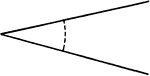### Angle

Illustration that can be used to show the definition of an angle - an opening between two lines that…### Bisected Angle

Illustration to show that if an angle is bisected, and if a line drawn through the vertex perpendicular…### Bisected Angles

Illustration to show that the bisectors of two supplementary adjacent angles are perpendicular to each…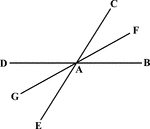### Bisected Angle

Illustration to show that the bisector of one of two vertical angles bisects the other.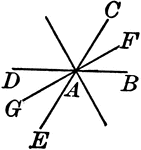### Bisected Angles

Illustration to show that the bisector of two pairs of vertical angles formed by two intersecting lines…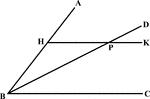### Angle Bisector Drawn Parallel to A Side

Illustration to show that if through any point in the bisector of an angle a line is drawn parallel…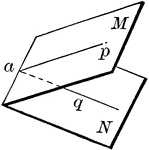### Dihedral Angle

A dihedral angle is the opening between two intersecting planes.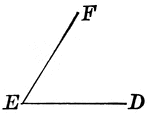### Plane Angle

An illustration of a plane angle with vertex E and sides EF and ED.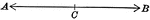### Straight Angle

An illustration showing a straight angle. When the sides of an angle extend in opposite directions,…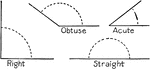### Angles

Four types of angles: right, obtuse, acute, and straight.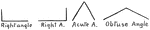### Angles

Showing different types of angles: right, acute, and obtuse.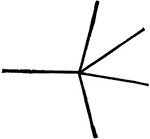### 360° Sum of Angles

Illustration showing that the sum of all the angles about a point equals 360°.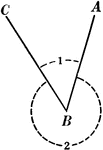### Acute and Reflex Angles

Illustration showing two positive angles; angle 1 being the acute angle and angle 2 being the reflex…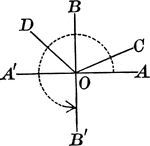### Acute, Obtuse, Straight, Right, And Reflex Angles

An illustration showing acute, obtuse, straight, right, and reflex angles.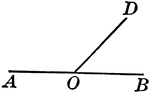An illustration of two angles that are adjacent. They have the same vertex and a common side between…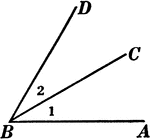Angles 1 and 2 are adjacent angles. Two angles with a common vertex and a common side between them are…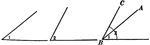### Difference of Angles

Illustration showing that the difference between angle 1 and angle 2 is angle ABC.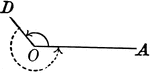### Obtuse And Reflex Angles

An illustration showing an angle the is greater than a right angle and less than a straight angle. This…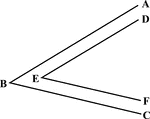### Angles With Parallel Sides

Illustration of angles with parallel corresponding sides.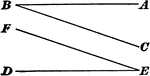### Angles With Parallel Sides

Illustration of angles with parallel sides going in the opposite direction.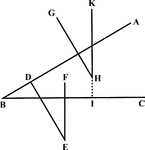### Angles With Parallel Sides

Illustration of angles with parallel sides. "GH is parallel to and lies in the same direction as DE,…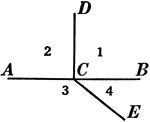### Relationships Between 4 Angles

Illustration showing four angles that can be used to define different relationships, such as adjacent,…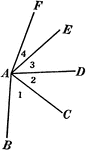### Relationships Between 4 Angles

Illustration showing four angles that can be used to define different relationships, such as adjacent,…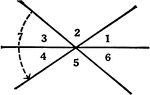### Relationships Between 6 Angles

Illustration showing six angles that can be used to define different relationships, such as adjacent,…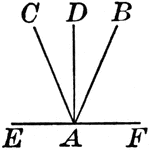### Angles With Same Vertex

An illustration of angles with the same vertex made up of 4 lines.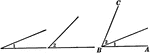### Sum of Angles

Illustration showing that the sum of angle 1 and angle 2 is angle ABC.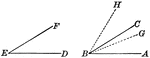### Superposition of Angles

Illustration of the superposition of two angles to compare magnitudes of the same kind.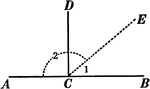### Supplementary and Right Angles

Illustration showing angles 1 and 2 are supplementary and angles ACD and DCB are supplementary. Also,…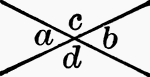### Vertical Angles

Vertical angles are when two angles have the same vertex, and the sides of the one are prolongations…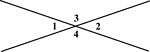### Vertical Angles

Illustration showing that angles 1 and 2 are vertical and angles 3 and 4 are vertical.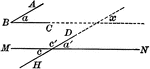### Angles With Parallel Sides

Illustration of two angles whose sides are parallel, each to each, to show they are either equal or…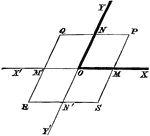### Oblique Axes

Angles on a modified coordinate plane.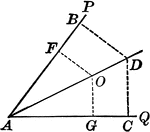### Angle Bisector

Illustration showing that the bisector of a given angle is the locus of points equidistant from the…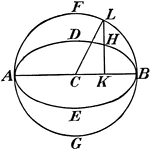### Eccentric Angle

"In geometry, an angle connected with an ellipse and defined as ... angle BCL, reckoned from one determinate…